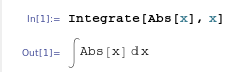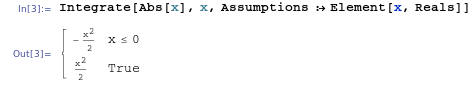## Integrating Abs(x) with Mathematica

February 17th, 2010 | Categories: general math, math software, mathematica, Wolfram Alpha | Tags:

Someone recently emailed me to say that they thought Mathematica sucked because it couldn’t integrate abs(x) where abs stands for absolute value.  The result he was expecting wasWhen you try to do this in Mathematica 7.0.1, it appears that it simply can’t do it. The command

Integrate[Abs[x], x]

just returns the integral unevaluatedI’ve come across this issue before and many people assume that Mathematica is just stupid…after all it appears that it can’t even do an integral expected of a high school student. Well, the issue is that Mathematica is not a high school student and it assumes that x is a complex variable. For complex x, this indefinite integral doesn’t have a solution!

So, let’s tell Mathematica that x is real

Integrate[Abs[x], x, Assumptions :> Element[x, Reals]]which is Mathematica’s way of saying that the answer is -x^2/2 for x<=0 and x^2/2 otherwise, i.e. when x>0. It’s not quite in the form we were originally expecting but a moments thought should convince you that they are the same thing.

Interestingly, it seems that Wolfram Alpha guesses that you probably mean real x since it just evaluates the integral of abs(x) directly. It does, however, give the result in yet another form: in terms of the signum function, sgn(x):A couple of weeks ago I am pretty sure that Wolfram Alpha gave exactly the same result as Mathematica 7.0.1 so I wonder if they have quietly upgraded the back-end Kernel of Wolfram Alpha.  Perhaps this is how Mathematica version 8 will evaluate this result?

1. I came across something similar just a couple of days ago! Any news about Mathematica 8, release dates in particular ;-) !?

2. Hi Sander

I know as much/little about Mathematica 8 as you do I’m afraid.

Cheers,
Mike

3. Thank you. Thank you. Thank you. Thank you very much for the Tips. That totally just saved me today. It saved me so much time from taking the integral of a nasty function :-D

4. I need help…. why Mathematica calculate this integral:

Integrate[ (-2 \[Pi] t) f[\[Tau] – \[ImaginaryI] t], {t, -\[Infinity], \[Infinity]}]

but not this:

Integrate[ (-2 \[Pi] Abs[t]) f[\[Tau] – \[ImaginaryI] t], {t, -\[Infinity], \[Infinity]},
Assumptions -> Element[t, Reals]]

if I’ve said that t is Real?

5. I have to say that things like this– the fact that Mathematic can’t solve very simple problems because it wants to do very hard problems– would be something I’d call “fail”.

Mathematica’s lack of solution may be technically accurate, but it’s useless.

6. Hi Geoffrey

So do you think that technically inaccurate solutions are more useful? Why would I want a wrong answer?

Mathematica gave the solution we expect when we posed the problem to it correctly which is exactly the behaviour I want. You may argue that it should behave as alpha does and volunteer the result that assumes all variables are real even if we don’t ask it to. This would be a bad idea in my opinion since the extra logic required would slow down integral evaluation.

7. OK, so I know a bit more about Mathematica 8 now ;)

http://www.walkingrandomly.com/?p=2933

8. Actually, a more graceful and readable way to do this is:

Assuming[Element[x,Reals], Integrate[Abs[x], x]]

It looks really sexy in TraditionalForm, but unfortunately doesn’t “paste” well:

Assuming[x\[Element]\[DoubleStruckCapitalR],\[Integral]\[LeftBracketingBar]x\[RightBracketingBar]\[DifferentialD]x]

9. We had identical discussions with my friend Olda – about calculations such as

Re[(-1)^(1/3)]

We actually started with much more complex an expression, but the thing above is what it boils down to.

Mathematica returns +1/2. Of course, elementary school students would prefer the answer -1. That’s because they choose the real value of the third root. However, it’s more natural to choose the “first complex” third root, as Mathematica does.

In my opinion, it’s very sensible that Mathematica behaves the way it does – tries to do thing analytically which strictly speaking means in terms of analytic functions of complex variables. The absolute value is not an analytic function, so one shouldn’t try to integrate it in the general way – in complex variables.

In fact, even in the real realm, it’s very contrived to “integrate the absolute value”. It’s very clear that this is just a shortcut to do a more awkward sequence of steps. Integrating of the absolute value in the real variables may sound natural to people who invent stupid exercises for math textbooks – but it is not natural in maths or science.

Mathematica behaves very sensibly. It’s the people who don’t understand the depth of the behavior who should learn new things. By the way, in most cases, the usage of complex numbers *adds* solutions to problems that wouldn’t have real solutions. That’s why complex numbers were invented in the first place.